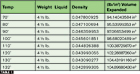Table 1.
[Editor’s Note: The analytical mind of today’s apprentice students is often underappreciated. The following article was motivated by a technical document that was read by an HVACR apprenticeship student, Sylvester Lipscombe, at the Federal Correctional Center in Petersburg, VA. With the assistance of his HVACR supervisor, Lowell Brooks, Lipscombe developed the following technical article that expands upon the material in the original document and includes extensive formulas and calculations.]

The issue of hydrostatic pressure in service cylinders is of concern to service technicians. It is a topic that has been covered in print from time to time, including in a service manual published several years ago. This article takes that material from the service manual and explains it in a bit more detail, focusing on the calculations involved.

The original article referred to above was published in Refrigeration Service Engineers Society’s (RSES’) RSES Service Application Manual (SAM)Form-Number 620-05 - Section 2 titled “Principles Of Refrigeration - Part 1 Subsection: Expansion And Contraction.” The following is the excerpt:

In the case of liquids, we are usually more interested in the increase in volume, or cubical expansion, than in the increase in just one dimension. We are well aware what can happen if a refrigerant cylinder is completely filled with liquid refrigerant in a cool room and is then allowed to become warmer. The liquid expands, and since the steel cylinder does not expand at the same rate, the cylinder bursts, not only ruining the cylinder and losing its contents - but more important, perhaps seriously injuring someone.

For example, a Refrigerant-12 [R-12] service cylinder has an internal volume of about 105 cubic inches. Suppose that we fill it entirely full of liquid R-12 leaving no room for expansion and that the liquid is at 70°F when we fill the cylinder. The 105 cu. in. of R-12 at 70° weighs 5 lbs. We put this completely filled cylinder into the truck.

The cylinder and R-12 warm up. When they get to 80°, the R-12 has expanded to a little over 106 cu. in. but the cylinder has expanded very little. Liquid R-12 can be compressed so little that it is almost negligible. When it expands, it exerts a very high hydrostatic or hydraulic pressure against the inner walls of the cylinder, probably about 1,000 pounds per square inch [psi] instead of the 70 pounds per psi of this same R-12 at 70°. The cylinder was built to withstand only about 450 to 500 pounds psi so it bursts.

Some cylinders are equipped with pressure relief valves, which open automatically when a pre-determined maximum pressure is reached, and close when some of the pressure is purged out.

Suppose that we put only 4 1/2 pounds of R-12 at 70° into the cylinder. It will take up only 94 cu. in., leaving 11 cu. in. for expansion. The cylinder would then have to warm up to 133° before the 94 cu. in. of R-12 would have expanded to 105 cu. in. and again filled the cylinder entirely full of liquid. So the cylinder would be safe up to 133°.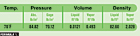Formula 1.
So how do we calculate the amount of liquid R-12 in a service cylinder with a volume of 105 cubic inches that is capable of holding at 70°? First, we need to refer to a Table on R-12 (Dichloroflouromethane) Saturated Properties (IP Units) and reference the properties given for 70°. What we would find is shown in Formula 1.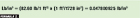Formula 2.
This information is necessary for determining how much liquid R-12 the cylinder would contain at 70°. And since the cylinder in the given example contains a volume of 105 cubic inches, we must convert the density of the liquid given in cubic feet to cubic inches. (See Formula 2.)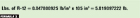Formula 3.
Now, since we know that the density of the R-12 is approximately 0.0478 pounds per cubic inch, we multiply this density by the volume of the service cylinder - which is 105 cubic inches - to obtain the weight of liquid R-12 that will completely fill this cylinder. (See Formula 3.)Formula 4.
Now, what happens if this R-12 cylinder is placed in a service van and the temperature rises to 80°? Again, referring to the Table on R-12, we obtain the following properties at 80°. (See Formula 4.)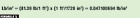Formula 5.
First, we must find the density of liquid R-12 at 80° and convert the density of the liquid from pounds per cubic feet to pounds per cubic inch. (See Formula 5.)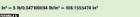Formula 6.
At this point, we see that at 80°, liquid R-12 has a density of approximately 0.0471 pounds per cubic inch. When we divide this density by the 5 pounds of R-12 in the cylinder, we obtain the volume needed to contain it at 80°. (See Formula 6.)

So we have proven that 5 pounds of R-12 expands to a volume of approximately 106.16 cubic inches at 80°. And at this temperature, the hydrostatic pressure exerted on the vessel will far exceed the 70.12 psig at 70° and the 84.06 psig at 80°.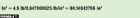Formula 7.
Finally, what happens if this cylinder is filled with 4-1/2 pounds of liquid R-12 at 70°? We already know from previous calculations that at 70°, the liquid R-12 has a density of approximately 0.0478 pounds per cubic inches. To determine the volume needed to contain the liquid R-12 at this temperature, we divide the 4-1/2 pounds of R-12 by the density of the liquid at 70°. (see Formula 7.)

Now we see that at 70°, the 4-1/2 lbs. of liquid R-12 requires a volume of only approximately 94.14 cubic inches as compared to the 105 cubic inches required for the 5 pounds of liquid R-12. And if we continue to chart the properties of the 4-1/2 lbs. of liquid R-12, we will arrive at the temperature in which this amount of liquid reaches a volume of 105 cubic inches (See Table 1).

At this point, we have proven that it will take approximately 133° for 4-1/2 lbs. of R-12 to reach a volume of 105 cubic inches. This also shows why most cylinders are manufactured at a design temperature of 130° with a fill capacity of approximately 80 percent.

Publication Date:09/24/2007